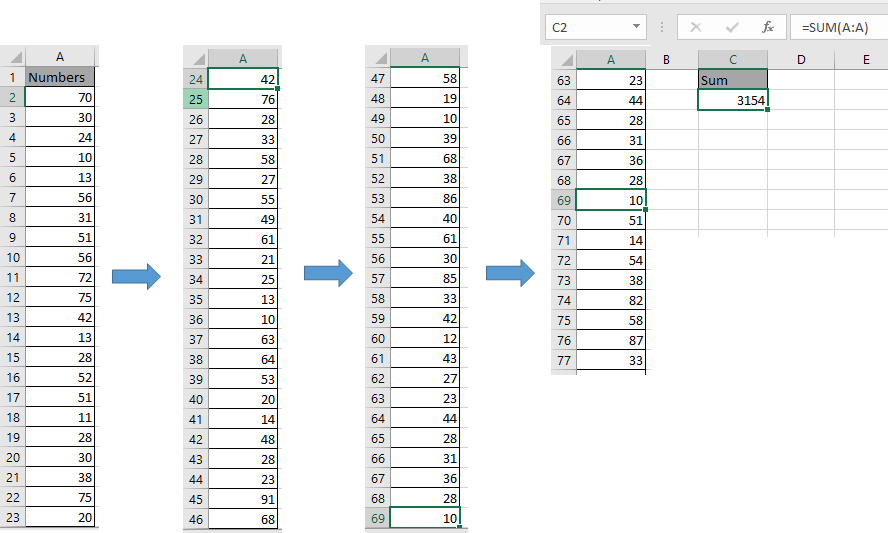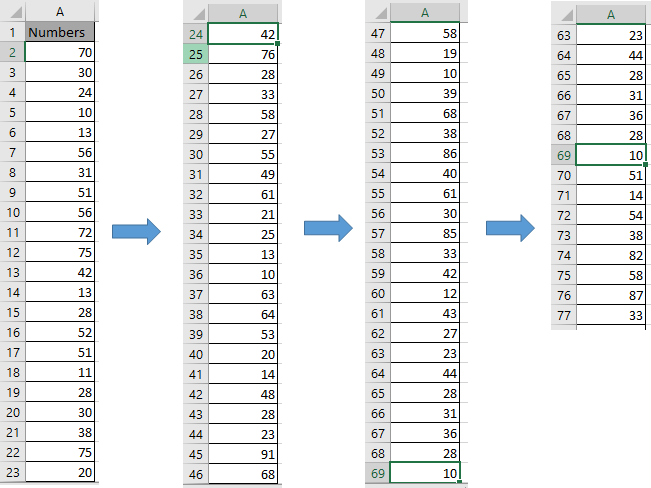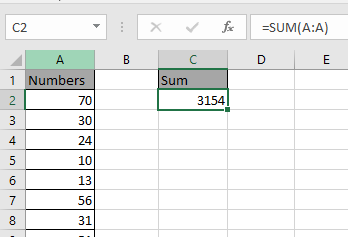# How to Sum across entire column in ExcelIn this article, we will learn How to Sum across an entire column in Excel.

SUM entire column

In Excel, working with array reference and need to access the whole column where you know empty cells are not interrupted. Like you can use SUM, COUNT, MAX function but cannot use AVERAGE or MIN function

SUM entire column Formula in Excel

SUM function adds up all the values. Here the whole column is

Syntax:

 =SUM(col : col)

Column numbers are indexed as A, B, C goes on. Like C:C when input numbers are in the Z column.

Example :

All of these might be confusing to understand. Let's understand how to use the function using an example. Here we have 76 numbers in column A.Here we need to sum the values present in this column their would two methods

Use the formula

 =SUM(A2:A77) OR =SUM(A:A)Sum function adds up the numbers in the list and returns the Sum.

Here are all the observational notes using the formula in Excel.
Notes :

1. The formula only works with only to look up the exact match.
2. The SUMPRODUCT function considers non-numeric values as 0s.
3. The SUMPRODUCT function considers logic value TRUE as 1 and False as 0.
4. The argument array must be the same size else the function returns an error.
5. SUMPRODUCT function returns the value corresponding to the TRUE values in the returned array after taking individual products in the corresponding array.
6. Operators like equals to ( = ), less than equal to ( <= ), greater than ( > ) or not equals to ( <> ) can be performed within a formula applied, with numbers only.

Hope this article about How to Sum across the entire column in Excel is explanatory. Find more articles on calculating values and related Excel formulas here. If you liked our blogs, share it with your friends on Facebook. And also you can follow us on Twitter and Facebook. We would love to hear from you, do let us know how we can improve, complement or innovate our work and make it better for you. Write to us at info@exceltip.com.

Related Articles :

Sum by OFFSET groups in Rows and Columns : The OFFSET function can be used to sum group of cells dynamically. These groups can be anywhere in the sheet.

SUM if date is between : Returns the SUM of values between given dates or period in excel.

Sum if date is greater than given date: Returns the SUM of values after the given date or period in excel.

2 Ways to Sum by Month in Excel: Returns the SUM of values within a given specific month in excel.

How to Sum Multiple Columns with Condition: Returns the SUM of values across multiple columns having condition in excel

Use INDEX and MATCH to Lookup Value : The INDEX-MATCH formula is used to lookup dynamically and precisely a value in a given table.  This is an alternative to the VLOOKUP function and it overcomes the shortcomings of the VLOOKUP function.

How to use wildcards in excel : Count cells matching phrases using the wildcards in excel.

Popular Articles :

How to use the IF Function in Excel : The IF statement in Excel checks the condition and returns a specific value if the condition is TRUE or returns another specific value if FALSE.

How to use the VLOOKUP Function in Excel : This is one of the most used and popular functions of excel that is used to lookup value from different ranges and sheets.

How to use the SUMIF Function in Excel : This is another dashboard essential function. This helps you sum up values on specific conditions.

How to use the COUNTIF Function in Excel : Count values with conditions using this amazing function. You don't need to filter your data to count specific values. Countif function is essential to prepare your dashboard.

Terms and Conditions of use

The applications/code on this site are distributed as is and without warranties or liability. In no event shall the owner of the copyrights, or the authors of the applications/code be liable for any loss of profit, any problems or any damage resulting from the use or evaluation of the applications/code.